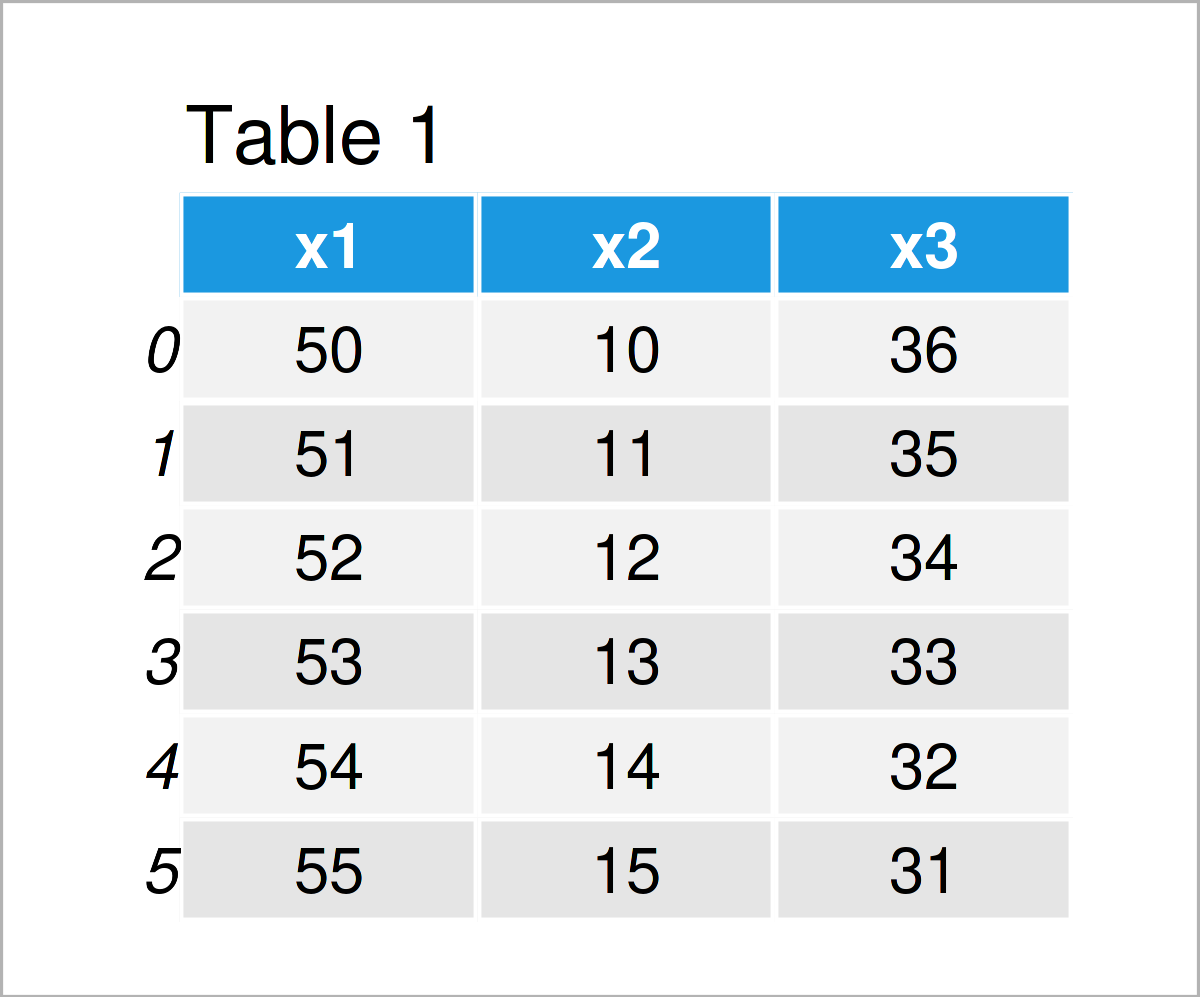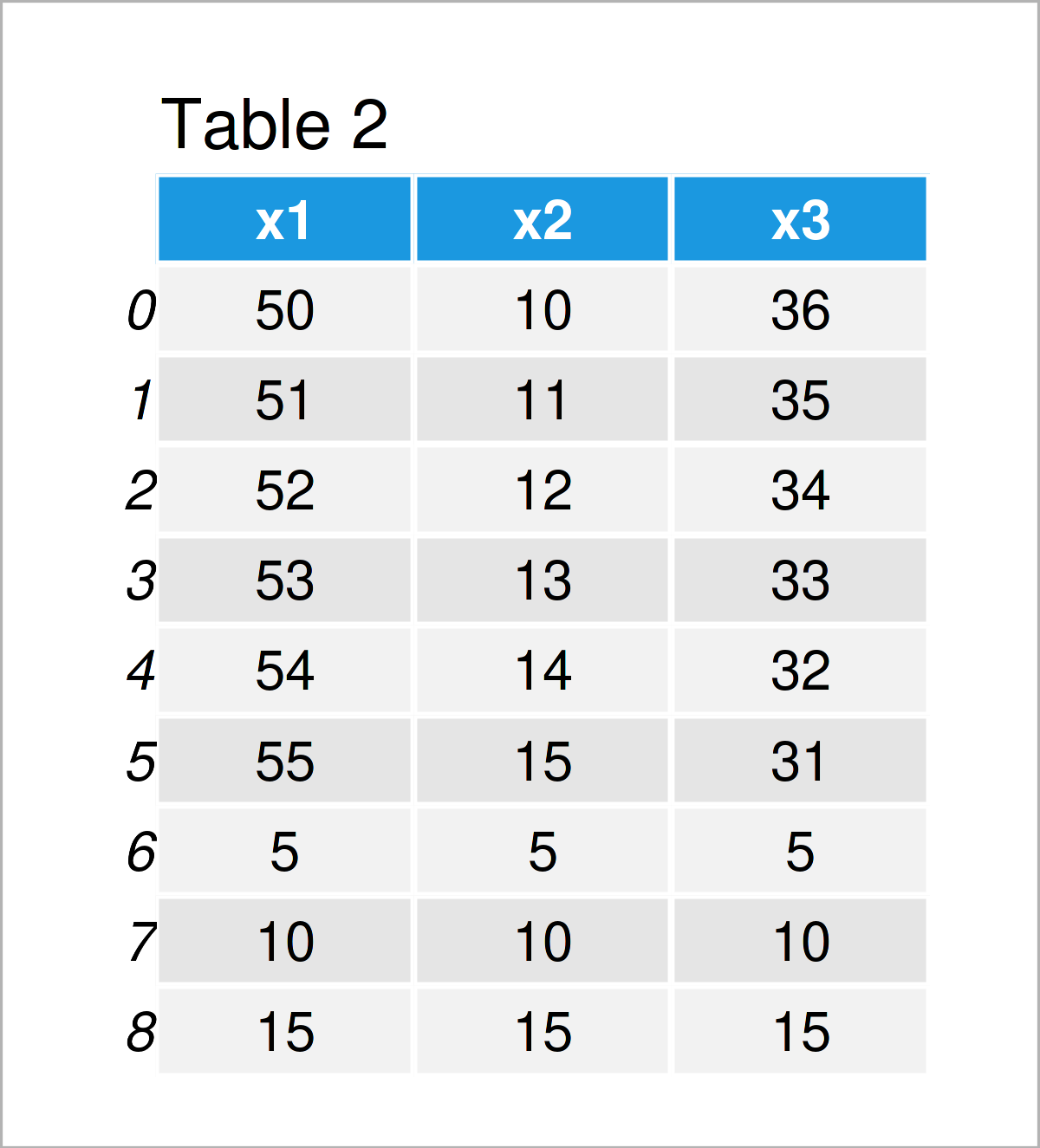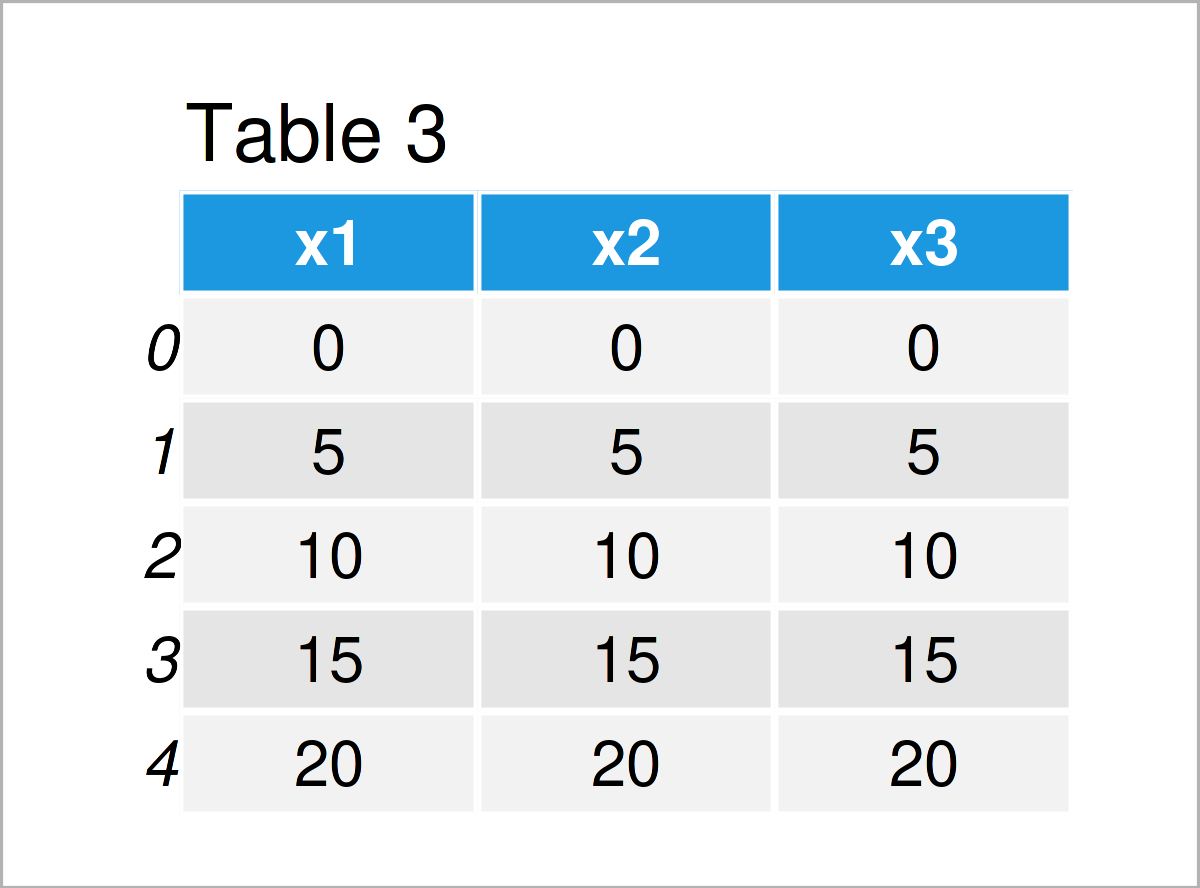# Append Rows to pandas DataFrame in Loop in Python (2 Examples)

In this post, I’ll illustrate how to add new rows to a pandas DataFrame using a for loop in the Python programming language.

## Example 1: Append Rows to pandas DataFrame within for Loop

The following Python syntax illustrates how to add new rows at the bottom of an existing pandas DataFrame within a for loop.

We first have to load the pandas library:

`import pandas as pd                                 # Import pandas library in Python`

In the next step, we can create an exemplifying pandas DataFrame for this example:

```data1 = pd.DataFrame({'x1':range(50, 56),           # Create pandas DataFrame
'x2':range(10, 16),
'x3':range(36, 30, - 1)})
print(data1)                                        # Print pandas DataFrame```As you can see in Table 1, we have created a pandas data set with three columns and six rows.

Now, we can use a for loop to add certain values at the tail of our data set. In this specific example, we’ll add the running index i times the value five.

Let’s do this:

```for i in range(1, 4):                               # Append rows within for loop
data1.loc[len(data1)] = i * 5
print(data1)                                        # Print updated DataFrame```By running the previous Python programming code, we have created Table 2, i.e. another pandas DataFrame containing the values of our input data set plus three new rows that have been concatenated within a for loop.

## Example 2: Append Rows to Empty pandas DataFrame within for Loop

In Example 1, I have explained how to combine an already existing pandas DataFrame with new rows created in a for loop.

In this section, I’ll demonstrate how to use a loop to build up a new data set from scratch.

Let’s first create an empty pandas DataFrame:

```data2 = pd.DataFrame(columns = ['x1', 'x2', 'x3'])  # Create empty DataFrame with column names
print(data2)                                        # Print empty DataFrame with column names
# Empty DataFrame
# Columns: [x1, x2, x3]
# Index: []```

In the next step, we can use a for loop to consecutively append new rows to our empty DataFrame:

```for i in range(5):                                  # Append rows within for loop
data2.loc[len(data2)] = i * 5
print(data2)                                        # Print updated DataFrame```As shown in Table 3, we have created a new pandas DataFrame where all values have been combined, filled in, and stacked within a for loop.

## Video & Further Resources

Do you want to know more about the addition of new rows to a pandas DataFrame within a loop? Then I recommend having a look at the following video on my YouTube channel. I show the Python programming codes of this article in the video instruction:

Furthermore, you may want to have a look at the related tutorials on www.statisticsglobe.com. Some other articles can be found below.

At this point you should have learned how to append new rows to a pandas DataFrame within a for loop in the Python programming language. In case you have any additional questions, tell me about it in the comments section.

Subscribe to the Statistics Globe Newsletter